﻿ Comparison between the Vector Control Based on Anti-saturation Capability with the Sensorless Vector Control in PMSM

### Comparison between the Vector Control Based on Anti-saturation Capability with the Sensorless Vector...OPEN ACCESSPEER-REVIEWED

## Comparison between the Vector Control Based on Anti-saturation Capability with the Sensorless Vector Control in PMSM

Behzad Salmani1, Fariborz Lohrabi Pour2,, Mohammad Bagher Bana Sharifian1

1Electrical and Electronic Department, Tabriz University, Tabriz, Iran

2Electrical and Electronic Department, Isfahan University, Isfahan, Iran

### Abstract

This paper investigates a comparison between the sensorless vector control method based on MRAS (Model Reference Adaptive System) using SVPWM (Space Vector Modulation) and the control method of the PMSM (Permanent Magnet Synchronous Motor) Based on anti-saturation current controller. MRAS algorithm is based on comparison between the estimators. The error between the estimated quantities obtained by the two models is used to evaluate the rotor speed that started in order to create an optimum vector control method. This method is compared with vector control based on anti-saturation current control block. That created in order to reduce the ripple in vector control current. These two control method were simulated in MATLAB and after comparing the current THD(Total Harmonic Distortion) of the two methods, it was observed the vector control based on anti-saturation block with shaft encoder has bigger volume, less current ripple and more cost than sensorless method.

### At a glance: Figures

12345
Prev Next

• Salmani, Behzad, Fariborz Lohrabi Pour, and Mohammad Bagher Bana Sharifian. "Comparison between the Vector Control Based on Anti-saturation Capability with the Sensorless Vector Control in PMSM." American Journal of Electrical and Electronic Engineering 2.1 (2014): 11-16.
• Salmani, B. , Pour, F. L. , & Sharifian, M. B. B. (2014). Comparison between the Vector Control Based on Anti-saturation Capability with the Sensorless Vector Control in PMSM. American Journal of Electrical and Electronic Engineering, 2(1), 11-16.
• Salmani, Behzad, Fariborz Lohrabi Pour, and Mohammad Bagher Bana Sharifian. "Comparison between the Vector Control Based on Anti-saturation Capability with the Sensorless Vector Control in PMSM." American Journal of Electrical and Electronic Engineering 2, no. 1 (2014): 11-16.

 Import into BibTeX Import into EndNote Import into RefMan Import into RefWorks

### 1. Introduction

Permanent magnet synchronous machines (PMSM) with regard to their many features, are widely used in a lot of industries, it is the main competitor of the induction motor in industrial equipment. The main reason for using this motor is usage of a permanent magnet excitation instead of stimulation winding that has made high efficiency motors.

On the other hand usage of the permanent magnets in this motor reduces the rotor weight and size in the same power output range of other motors. So the motors will have a higher power density also the excitation system copper losses have been removed and efficiency has increased. Heat is generated in the stator and it’s easier to pass it, also construction defect or damaged from mechanical failure happens very rarely in permanent magnets and this issue increases the reliability of this motor rather than the others. The main problem in permanent magnet motors is high cost of permanent magnets that are used in these motors.According to rates that listed for PMSMs and more application for these motors the circuit of these motors must be designed with optimum efficiency and cost, and also the main control parameters in these motors such as speed, current and torque should be controlled well and with stability. Using an appropriate method for controlling the motors can create a complete collection. Several methods such as vector control and direct control had been suggested for controlling these motors that each had advantages and disadvantages. Here is a comparison between two methods of vector control and sensorless vector control that each of these methods has its own advantages and disadvantages and the goal does not undermine any of the methods [1, 2, 3, 4, 5, 6].

### 2. Sensorless Vector Control

In recent years extensive studies about the speed and position estimation is performed. In this method a new sensorless controller based on MRAS (Model Reference Adaptive System) is presented that this method uses a state observer with the current error feedback and the magnetic flux model that the two models for estimating the back-EMF can be observed. Speed and torque control of permanent magnet synchronous motor is usually attained by application of speed or position sensor. However, speed and position sensors require the additional mounting space, reduce the reliability in harsh environments and increase the cost of motor.

This paper proposed an optimized sensorless vector control method based on MRAS using space vector modulation. The MRAS algorithm is based on the comparison between the outputs of two estimators. The error between the estimated quantities obtained by the two models is used to drive a suitable adaption mechanism which generates the estimated rotor speed. The changes in the control method leads to removing speed and position sensor which instead we can use other methods such as state equation, kalman-filter, luenberger filter, sliding mode control, out standing the impact, artificial intelligence, direct control of flux and torque. And also, in order to do a proper switching we can use the space vector control that not alone gives an optimal voltage vector based on passive and active voltage vectors and an optimal switching, it also is resulted in reduction in the steady-state flux and torque ripple in the control system compared to conventional methods. Block diagram of the control method shown in Figure 1.

Figure 1. Block diagram of the sensorless vector control mehod based on MRAS estimator and space vector modulation (SVPWM)
2.1. Mathematical Modeling of the Motor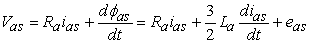(1)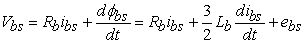(2)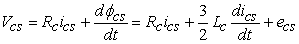(3)

Where back-EMF of the phase winding induced from the flux of the permanent magnet is such as follows: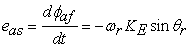(4)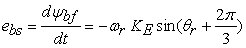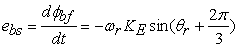(5)

Where and KE is the back-EMF constant .

From (1)-(3), α- and β–axis voltage equations in the stationary reference frame fixed to the stator may be expressed as: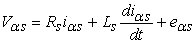(6)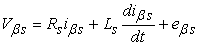(7)

Where Rs = Ra and Ls =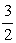La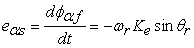(8)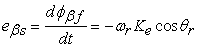(9)

Where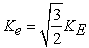From (1)-(3), d- and q-axis voltage equations in the reference frame with the rotating speed of wr may be expressed as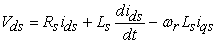(10)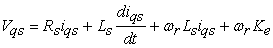(11)

The electromagnetic torque in the rotor reference frame may be expressed as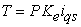(12)

Where P is the number of poles

And at the end, the mechanical equation of a PMSM may be expressed as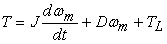(13)

Where J is the inertia coefficient and D is the friction coefficient, wm is the mechanical speed of the rotor and TL is the load torque.

2.2. Sensorless Control Based on MRAS

In this method, can be observed a new sensorless control based on MRAS. In general the MRAS algorithm is based on comparison between the output of two estimators, the error between the estimated quantities obtained by the two models is used to drive a suitable adaption mechanism which generates the estimated rotor speed. The MRAS algorithm is well-known in the sensorless control of an induction motor and has been proved to be effective and physically clear. This method is using the state observer model with the current error feedback and the magnet flux model as two models for the back-EMF estimation [7, 8, 9].

2.3. State Observer Configuration

From (9) and (10), the state equations of the full order observer in the stationary reference frame may be expressed as: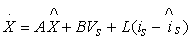(14)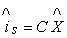(15)

Where ^ means the estimated value, L is the observer gain.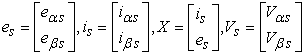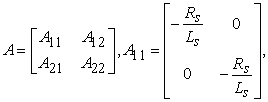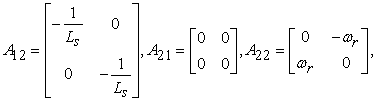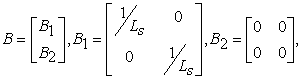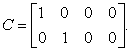In this method the estimated currents may be replaced by the measured currents, and the order of the observed states may be reduced and in here the reduced order observer which may be expressed as follows: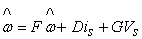(16)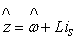(17)

Also we have a series of definition by the following: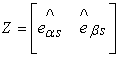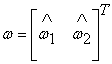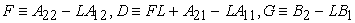Figure 2 shows the block diagram of the reduced order state observer for the back-EMF estimation.

2.4. MRAS Configuration

This method proposes a novel sensorless control algorithm based on the MRAS for the speed sensorless control of a PMSM. The proposed MRAS is using the state observer model of (17) and (18) and the magnet flux model of (9) and (10) as two models for the back-EMF estimation. The rotor speed is generated from the adaptation mechanism using the error between the estimated quantities obtained by the two models as follows: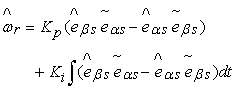(18)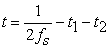Where K p and Ki are the gain constants,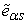and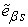are the estimated values of back-EMFs in the state observer model, andandare the estimated values of back-EMFs in the magnet flux model.

Figure 3 shows the block diagram of the proposed MRAS. The proposed MRAS algorithm has a robust performance through combining the state observer model and the magnet flux model.

2.5. Space Vector Modulation (SVPWM)

In this method a voltage vector with an appropriate time dividing between the voltage vectors and its neighbors (from V1 to V6 voltage vectors and two zero vectors (V0, V7)) is made. Actually in this method, average of the three Vi+1, Vi, V0 (or V7) vectors in a desires period are applied to the motor.

In this method, we need the voltage reference Vs* with fixed frequency fs2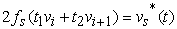(19)(20)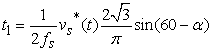(21)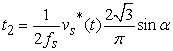(22)

To minimize the number of commutation for reference voltage space vector Vs* that is located in the first part of switching is done as follows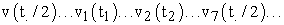switching order for all the odd sub cycle is like the above order and also switching order for even cycles is.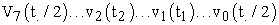The other switchings are obtained in the same way with imaging of the voltageon the six base voltages. Therefore on this basis we can obtain an appropriate lookup table with high precision for this method to determine the proper voltage vector from the three created voltage vector in each area .

### 3. Vector Control Based on Anti-saturation Current Control

In this method an advance dc indirect vector control based on lookup table and considering required constraints to prevent the current controller from saturation is presented To improve the problems of the permanent magnet To improve the problems of the permanent magnet synchronous motor (PMSM) vector control methods, a novel method based on the use of anti-saturation block is made which has been combined with vector control method. Saturation and temperature error will cause a difference between input and output. Generating the reference voltages from resulting currents will cause the lack of suitable coupling between the d and q axis. That for better differentiation between the two d and q axis’s variables decoupling is used .

Figure 4 is block diagram of the proposed method. also to avoid saturation of the current limiter we can use the stator current limiter and voltage inverter that at high speeds prevents saturation of these controllers and it can damp the error between the input and output, which the benefits of this method is lack flux and torque estimator and removing the hysteresis controller.

3.1. Necessary Limination to Prevent the Saturation of the Current Controller

The first limit of the current is the maximum current passing through the winding at steady state. The equation corresponding this limit in two-axis dq coordinate system is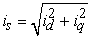(23)

The second limit is the inverter voltage which is a function of the motor speed. To obtain the boundries of this limit region we write the two-axis motor equations in the steady state dq coordinate system and obtained an equation as a function of iq and id currents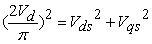(24)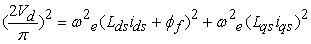(25)

The following equations are achieved by simplification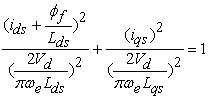(26)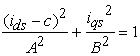(27)

Vd is the DC bus voltage above equation as a function of id and iq is an ellipse an example of this ellipse variations versus speed variations is shown in Figure 6.

It can be seen that the greater the speed the smaller the size of the ellipse. For speeds near infinity, the ellipse tends to a single point. Therefore at high speed the inverter can`t supply the motor voltage. Now, the motor current vector that it is the work point should be located inside the ellipse and circle common border that we talk about it. Till both limitations from limit of inverter voltage and winding current became satisfied. The strategy to limit the current vector and the novel idea to prevent saturation is that if the current vector is located outside the ellipse border, we`ll keep id constant and decrease iq till the current vector locates inside the ellipse. If by decrease iq till the current vector locates inside the ellipse. If by decreasing iq the current vector is located inside the ellipse but outside the circle. Applying these instructions, we can locate the current vector on a point other than the saturation points.

Today with applied the appropriate vector control to the motors, a wide range of speed and torque are achieved. In this control method is addition to providing stable and speed and excellent of accuray answer due to applied feedback to motor is possible to independent control of the flux and torque of the motor.In this method the control parameters are id and iq of the motor. With control the id, flux of the motor can be controlled and as a result speed of the motor can be controlled and with control the iq which is the component of the torque , output constant torque is adjustable [10, 11].

### 4. Simulation

Both control methods are compared in the following nominal values. Both motor nominal values are expressed in Table 1.

#### Table 1. Motor nominal valuesDownload asPowerPoint Slide

Veiw figureView current table in a new window

3phase currents are shown in Figure 9.

Figure 10 shows the speed response in the speed command of 1500 in the vector control based on anti-saturation current control

Figure 10. Speed response in the speed command of 1500 rpm in the vector control based on anti-saturation current control method

Also motor output current and torque in the torque command of 2 N.m in the vector control based on anti-saturation current control is as shown in Figure 11 and Figure 12.

For comparing the two vector control methods we calculate THD (Total Hamonic Distortion) of the current for the two methods in the speed command of 1500 rpm and the torque command of 2N.m, then we compare the two THD.

Figure 13 shows the single phase motor output current and the THD in the sensorless vector control method.

Figure 14 shows the single phase motor output current and the THD in the vector control based on anti-saturation current control method.

Figure 14. THD of the current in the vector control based on anti-saturation current control method (THD = 1.39%)

Vector control based on vector control based on anti-saturation current control has less ripple rather than the vector control method based on sensorless vector control.

### 5. Conclusions

In this paper a sensorless vector control method based on MRAS (Model Reference Adaptive System) using SVPWM (Space Vector Modulation) is compared with PMSM vector control based on anti-saturation current control method based on anti-saturation of the current controller. MRAS algorithm is based on comparing the two estimator output. The error between the estimated quantities obtained by the two models is used to drive a suitable adaptation mechanism which generates the estimated rotor speed. The other method is a vector control method with taking the anti-saturation current block for prevention the the PI controller from saturation. The goal does not undermine any of the methods, but the comparison is for creating an improved vector control methodor using a vector control method with less current ripple and less volume in different working conditions. From comparison of the THD for currents, we can conclude that however the sensorless method has lower cost and volume rather than the vector control based on anti-saturation current control method with shaft encoder, but it has more current ripple than the vector control based on anti-saturation current control method.

### References

  A. Sikorski, M. Korzeniewski, A. Ruszczyk M.P. Kazmierkowski, P. Antoniewicz, W. Kołomyjski, M. Jasinski, A Comparison of Properties of Direct Torqueand Flux Control Methods (DTC-SVM, DTC)DTC-2x2, DTFC-3A), International Conference on “Computer as a Tool” Warsaw, September 9-12EUROCON 2007.In article  H. Zhu, X. Xiao, Y. Li, PI type dynamic decoupling control scheme for PMSM high speed operation,, Applied Power Electronics Conference and Exposition (APEC) ,2010 Twenty-Fifth Annual IEEE.In article  Ion Boldea S.A. Nasar, Electrical Drives Book (2005).In article  T. G. Habetler, F. Profumo, M. Pastorelli, and L. M. Tolbert, “Direct torque control of induction machines using space vector modulation”, IEEE Transactions on Industry Applications, Vol.28, No. 5, Sep/Oct 1992, pp.1045-1053.In article CrossRef  A. Sikorski and T. Citko, “Current controller reduced switching frequency for VS-PWM invertet used with AC motor drive application”,IEEE Transactions on Industrial Electronics, Vol. 45, No.5, Oct. 1998, pp. 792-801.In article CrossRef  M. Zelechowski, M.P. Kazmierkowski, F. Blaabjerg. Controller Design for Direct Torque Controlled Space Vector Modulated (DTC-SVM) Induction Motor Drives”, IEEE ISIE 2005, Dubrovnik, Croatia, pp. 951-95.In article  C. Schauder, "Adaptive Speed Identification for Vector Control of Induction Motors without Rotational Transducers," IEEE Trans Ind Appl, Vol. 28, No. 5, pp. 1054-1061 (1992).In article CrossRef  Y. A. Kwon and D. W. Jin, "A Novel MRAS Based Speed Sensorless Control of Induction Motor," IEEE Proc IECON, Vol. , pp. 933-938 (1999).In article  S. Morimoto, H. Nakayama, and M. Sanada, “Sensorless output maximization control for variable-speed wind generation system using IPMSG,”IEEE Transactions on Industry Applications, vol. 41, no. 1, pp. 60-67, Jan/Feb 2005.In article CrossRef  C. Cavallaro, A.O. Tommaso, R. Miceli, and A. Raciti, “Efficiency enhancement of permanent magnet synchronous motor drives by online loss minimization approaches,” IEEE Transactions on Industry Applications, vol. 52, no. 4, pp. 1153-1160, August (2005).In article  J.S. Yim, S.K. Sul, B.H. Bae, N.R. Patel, and S. Hiti, “Modified current control schemes for high-performance permanent-magnet ac drives with low sampling to operating frequency ratio,” IEEE Trans. Industry Applications, vol. 45, no. 2, pp. 763-771, March/April (2009).In article